# Running your statistical test in SPSS

## How to run common statistical tests in SPSS and report them in APA style

This workbook will take you through how to run a variety of common statistical tests in SPSS. If you want to know more about how to choose your statistical test and how to set up your data then have a look at our post ‘Getting started with SPSS and calculating descriptive statistics’.

Tip: We cannot cover every test in this post. For help and support with other tests visit the maths and stats support service.

This post covers:

# 1. Running an independent samples t-test

An independent samples t-test is used to compare the means of a normally distributed scale dependent variable between two independent groups. For example, comparing test scores of men and women (where test scores are assumed to be continuous).

Here’s how to run it in SPSS (the example of test score and gender will be illustrated in brackets):

1. Select ‘Analyze’ from the top menu.
2. Choose ‘Compare Means’ and then ‘Independent-Samples T Test’.

3. Select your dependent variable (test score) and click on the top blue arrow to move it to the ‘Test Variable(s)’ box.

4. Then select your independent variable (gender) and click the second blue arrow to move it to the ‘Grouping Variable’ box.

5. Next click on ‘Define Groups’.

6. This will bring up the ‘Define Groups’ pop-up as seen below.

7. Enter the values representing the two groups of your independent variable (0 for Male and 1 for Female).

8. Click ‘Continue’ and then ‘OK’.

This will open up the output window with your results.

## Interpreting the output and reporting in APA style:

If the test for equal variances (circled in blue above) is greater than 0.05 then we can assume the variances are equal and read our results from the top row of the table. If the value is less than 0.05 then we can’t assume equal variances and we have to read our results from the bottom row of the table.

Reading along the top row of our table we can see that the test found that the mean difference of 0.378 wasn’t significant as the p-value, 0.792, is greater than 0.05.

We could report our results as follows:

An independent samples t-test was run to compare the test scores of males (M = 9.92, SD = 4.316) and females (M = 9.54, SD = 2.634). The difference, 0.378, 95% CI [-2.553, 3.309], was not statistically significant, t(23) = 0.267, p = 0.792 .

^back to contents

# 2. Running a Mann-Whitney U test

The Mann-Whitney U test is a non-parametric test to compare two independent groups of an ordinal dependent variable. This is very similar to the independent samples t-test but for the Mann-Whitney U we don’t need to assume that our dependent variable is normally distributed or continuous.

We will use the same example of comparing test scores between genders as we did for the independent samples t-test however this time we won’t assume that the test scores are continuous.

Here’s how to run it in SPSS:

1. Select ‘Analyze’ from the top menu.
2. Choose ‘Nonparametric Tests’, ‘Legacy Dialogs’ and then ‘2 Independent Samples’.

3. Select your dependent variable (test score) and click on the top blue arrow to move it to the ‘Test Variable List’ box.

4. Then select your independent variable (gender) and click the second blue arrow to move it to the ‘Grouping Variable’ box.

5. Next click on ‘Define Groups’.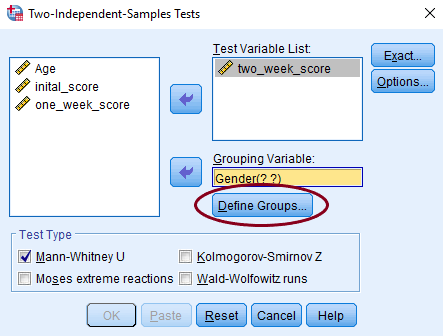Steps 3, 4 and 5 in running a Mann-Whitney U test

6. This will bring up the ‘Define Groups’ pop-up as seen below.

7. Enter the values representing the two groups of your independent variable (0 for Male and 1 for Female).

8. Click ‘Continue’.

9. Make sure ‘Mann-Whitney U’ is ticked in the ‘Test Type’ box.

10. Then click ‘OK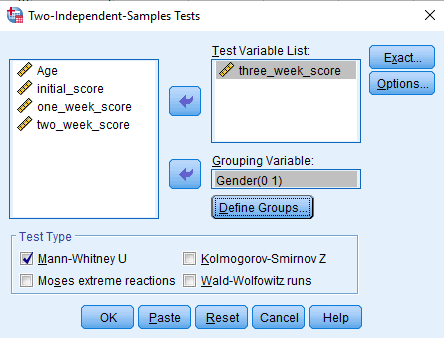Steps 7, 8 and 9 in running a Mann-Whitney U test

This will open up the output window with your results.

## Interpreting the output and reporting in APA style:

Looking at our ‘Test Statistics’ table we can see that there wasn’t a significant difference between the test scores of males and females because our p-value, 0.913, is greater than 0.05.

We could report our results as follows:

A Mann-Whitney U test was run to compare the test scores of males and females. The test indicated that the difference was not statistically significant, U(Nmale = 12, Nfemale = 13) = 76.00, z = -0.110, p = 0.913 .

^back to contents

# 3. Running a paired samples t-test

A paired samples t-test is used to compare means of two related observations of normally distributed, scale variables. For example, comparing how fast the same group of people ran the 100m in the semi-finals and the final of the Olympics.

Here’s how to run it in SPSS:

1. Select ‘Analyze’ from the top menu.
2. Choose ‘Compare Means’ and then ‘Paired-Samples T Test’.

3. Select the first observation of your dependent variable (100m final time) and click on the blue arrow to move it to the ‘Variable 1’ box.

4. Then select the second observation of your dependent variable (100m semi-final time) and click on the blue arrow again to move it to the ‘Variable 2’ box.

5. Then click ‘OK’.

This will open up the output window with your results.

## Interpreting the output and reporting in APA style:

Looking at our ‘Paired Samples Test’ table we can see that there wasn’t a significant difference between the 100m times of the runners in the semi-finals and final because our p-value, 0.723, is greater than 0.05.

We could report our results as follows:

Runners’ 100m times were compared in the semi-finals (M = 10.0810, SD = 0.58932) and the final (M = 10.1445, SD = 0.52087). A paired samples t-test indicated that the difference, 0.06350, 95% CI [-0.31184, 0.43884] was not statistically significant, t(15) = 0.361, p = 0.723.

^back to contents

# 4. Running a Wilcoxon signed rank sum test

The Wilcoxon signed rank sum test is a non-parametric test to compare two related observations. This is very similar to the paired t-test but we don’t need to assume that the difference between the two variables is normally distributed or continuous.

We will use the same example of comparing 100m times between the semi-finals and finals of the Olympics as we did for the paired samples t-test however this time we won’t assume that the differences are normally distributed.

Here’s how to run it in SPSS:

1. Select ‘Analyze’ from the top menu.
2. Choose ‘Nonparametric Tests’, ‘Legacy Dialogs’ and then ‘2 Related Samples’.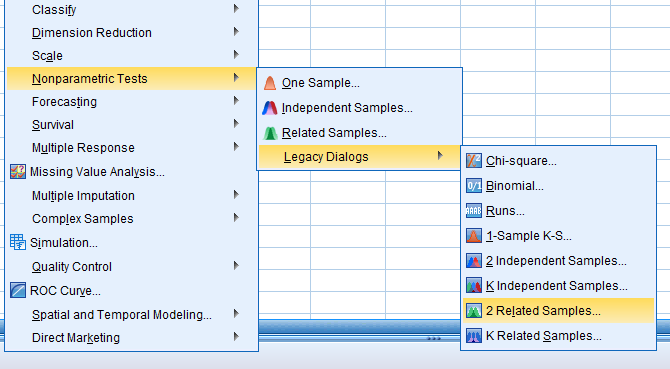Steps 1 and 2 in running a Wilcoxon signed rank sum test

3. Select the first observation of your dependent variable (100m final time) and click on the blue arrow to move it to the ‘Variable 1’ box.

4. Then select the second observation of your dependent variable (100m semi-final time) and click on the blue arrow again to move it to the ‘Variable 2’ box.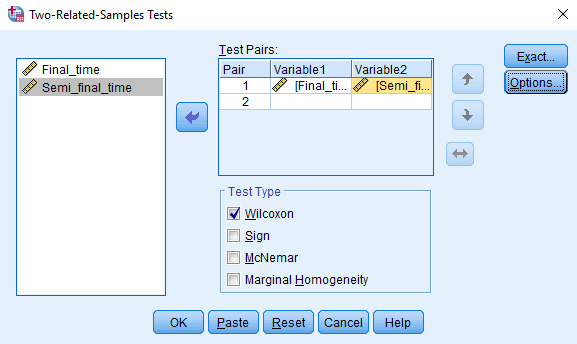After completing Steps 3 and 4 in running a Wilcoxon signed rank sum test

5. Click on the blue ‘Options’ button on the right hand side.

6. Tick the boxes for ‘Descriptive’ and ‘Quartiles’.

7. Then click ‘Continue’ and ‘OK’.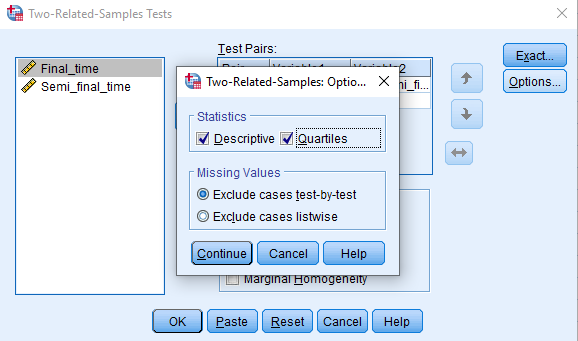Steps 6 and 7 in running a Wilcoxon signed rank sum test

This will open up the output window with your results.

## Interpreting the output and reporting in APA style:

Looking at our ‘Test Statistics’ table we can see that there wasn’t a significant difference between the 100m times of the runners in the semi-finals and final because our p-value, 0.796, is greater than 0.05.

Our test statistic, T, is the smaller value from the sum of positive ranks (63) and the sum of negative ranks (73).

We could report our results as follows:

Runners’ 100m times were compared in the semi-finals (Mdn = 10.1023) and the final (Mdn = 10.1758). A Wilcoxon signed-rank test indicated that the difference was not statistically significant, T = 63, z = -0.259, p = 0.796 .

^back to contents

# 5. Running a one-way ANOVA

A one-way ANOVA is used to compare differences in means of the normally distributed scale dependent variable across the levels of a categorical independent variable. For example, comparing the mean time to travel to work using different types of public transport.

Here’s how to run it in SPSS:

1. Select ‘Analyze’ from the top menu.
2. Choose ‘Compare Means’ and then ‘One-Way ANOVA’.

3. Select your dependent variable (time of commute) and click on the top blue arrow to move it to the ‘Dependent List’ box.

4. Then select your independent variable (mode of transport) and click the second blue arrow to move it to the ‘Factor’ box.

5. Next click on ‘Options’ on the right hand side, tick ‘Descriptive’ and click ‘Continue’.

6. Then click on ‘Post Hoc’ on the right hand side.

7. Tick the box next to ‘Tukey’.

8. Click ‘Continue’ and then ‘OK’.

This will open up the output window with your results.

## Interpreting the output and reporting in APA style:

Looking at our ‘ANOVA’ table we can see that there was a significant difference between the time taken to get to work by bus, train and bike because our p-value, 0.000, is less than 0.05. When our p-value is 0.000 we report it as p < 0.001 .

We could report our results as follows:

An ANOVA was run to examine the effect of mode of transport on time taken to get to work. The test indicated that mode of transport does have a statistically significant effect on time taken to get to work, F(2, 27) = 25.028, p < 0.001 .

This however doesn’t tell us where these differences are, just that there are some. To find out more about the differences we look at our Tukey’s post-hoc test in the ‘Multiple Comparisons’ table.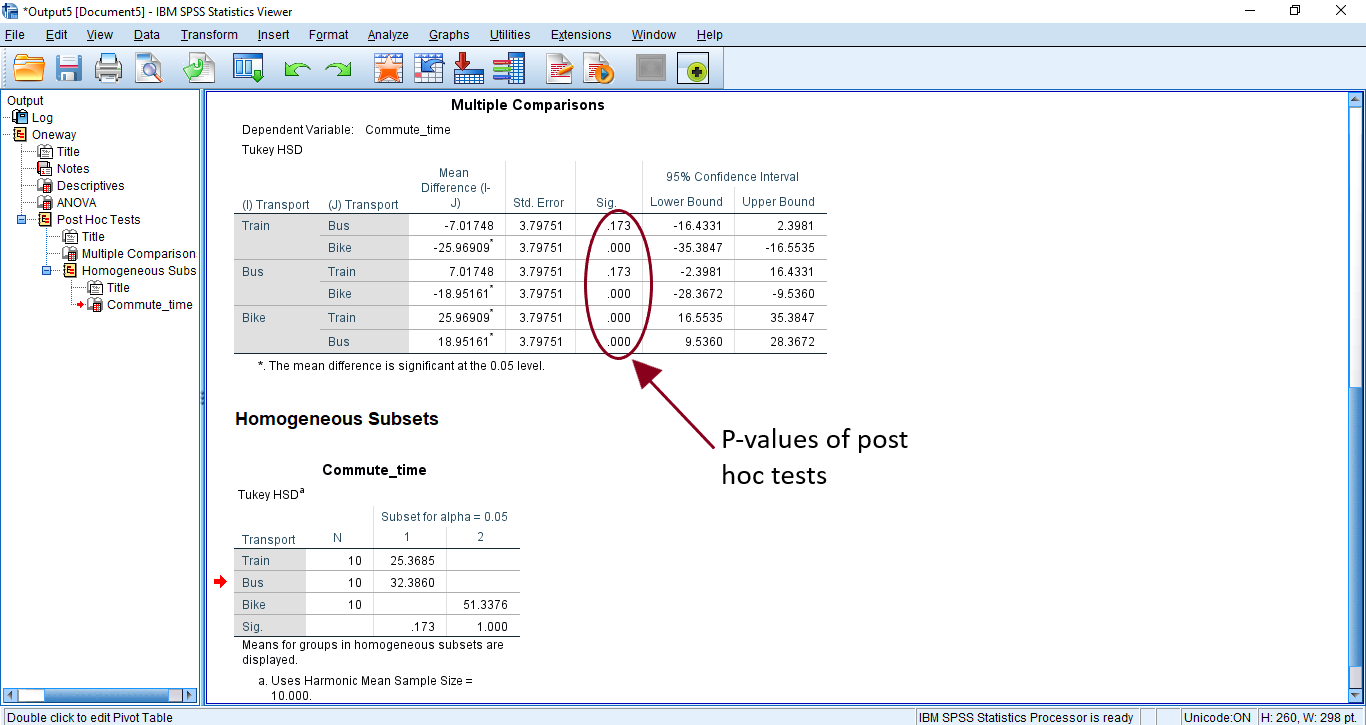SPSS output for the post hoc tests with key values labelled

Looking at our ‘Multiple Comparisons’ table we can see that there wasn’t a significant difference between travelling by train and by bus because our p-value, 0.173, is greater than 0.05. However, there is a significant difference between travelling by train and by bike as well as between travelling by bus and by bike.

We could report our results as follows:

Travelling by train, on average, decreased the time taken to get to work compared to travelling by bike. Post hoc comparisons using the Tukey HSD test revealed that this difference, 25.9690, 95% CI [16.5535, 35.3847], was statistically significant, p < 0.001. Travelling by bus also significantly reduced travel time by 18.9516 minutes, 95% CI [9.5360, 28.3672], p < 0.001 compared to travelling by bike. However, the difference in travel time, 7.0174, 95% CI [-2.3981, 16.4331] between travelling by train and travelling by bus and travelling by train was not statistically significant, p = 0.173 .

^back to contents

# 6. Running a Kruskal Wallis test

The Kruskal Wallis test is a non-parametric test that is used when we have an independent variable with two or more levels and an ordinal dependent variable. This is very similar to the one-way ANOVA but we don’t need to assume that the dependent variable is continuous or normally distributed.

We will use the same example of comparing commute times with different modes of transport as we did for the one-way ANOVA however this time we won’t assume that the times are normally distributed.

Here’s how to run it in SPSS:

1. Select ‘Analyze’ from the top menu.
2. Choose ‘Nonparametric Tests’, ‘Legacy Dialogs’ and then ‘K Independent Samples’.

3. Select your dependent variable (time of commute) and click on the top blue arrow to move it to the ‘Test Variable List’ box.

4. Then select your independent variable (mode of transport) and click the second blue arrow to move it to the ‘Grouping Variable’ box.

5. Next click on ‘Define Range’, enter the minimum and maximum values in your independent variable (0=train, 1=bus, 2=bike, so 0 and 2 are the min and max) and click ‘Continue’.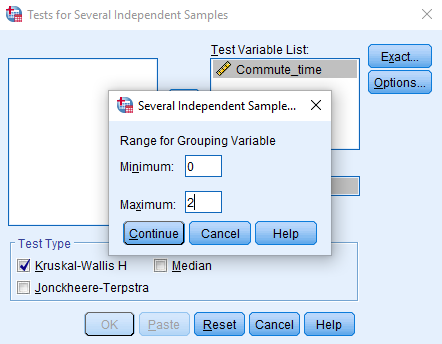Steps 3, 4 and 5 in running a Kruskal Wallis test

6. Make sure ‘Kruskal-Wallis H’ is ticked in the ‘Test Type’ box at the bottom.

7. Then click ‘OK

Tip: You will also need to calculate descriptive statistics of your dependent variable with your independent in the ‘Factor List’ box for reporting purposes. You can read about how to do that in our post ‘Choosing a test and calculating descriptive statistics’.

This will open up the output window with your results.

## Interpreting the output and reporting in APA style:

Looking at our ‘Test Statistics’ table we can see that there was a significant effect of mode of transport on time taken to get to work because our p-value, 0.000, is less than 0.05. When our p-value is 0.000 we report it as p < 0.001 .

We could report our results as follows:

A Kruskal Wallis test showed that mode of transport significantly affects the amount of time taken to get to work, H(2) = 24.581, p < 0.001.

If the Kruskal Wallis test is significant then we can run Mann-Whitney tests or a Dunn-Bonferroni post hoc method to find out which of the groups are significantly different.

^back to contents

# 7. Running a one-way repeated measures ANOVA

A repeated measures ANOVA is used when we have a categorical independent variable and a normally distributed, scale dependent variable that is measured at least twice for each subject. This is similar to the paired samples t-test but allows us to have more categories of our independent variable.

For example, we might want to look at how students test scores change as they are given the same test at the end of each school year.

Here’s how to run it in SPSS:

1. Select ‘Analyze’ from the top menu.
2. Choose ‘General Linear Model’ and then ‘Repeated Measures’.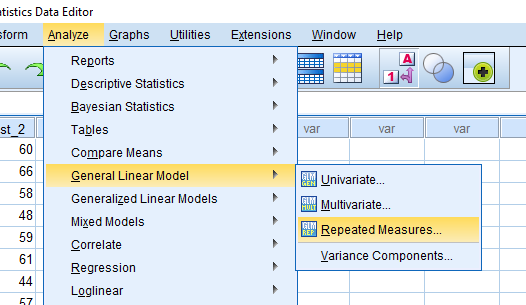Steps 1 and 2 in running a one-way repeated measures ANOVA

3. Change the ‘Within-Subject Factor Name’ to something relevant to your independent variable (time of test taken).

4. Enter the number of levels (in this case we have the tests taken once a year for 3 years).

6. Then add a useful ‘Measure Name’ which describes your dependent variable (test score).

7. Click ‘Add’ and then click ‘Define’.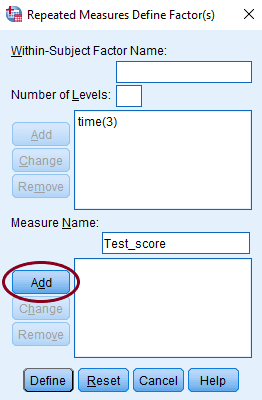Steps 3 to 7 in running a one-way repeated measures ANOVA

8. This opens up the repeated measures window.

9. Click the blue arrow to move your variables into the ‘Within-Subjects Variables’ box.

Tip: If they aren’t in chronological order then you can use the up and down blue arrows to re-order them.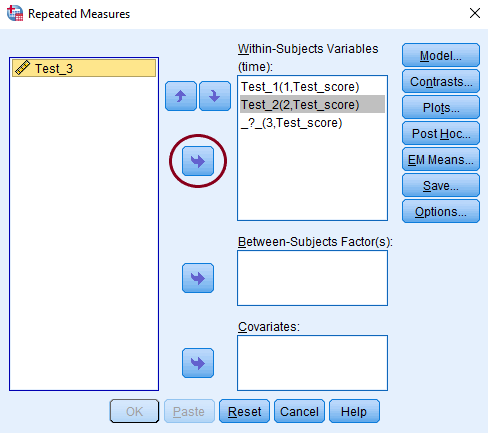Steps 8 and 9 in running a one-way repeated measures ANOVA

10. Select the ‘EM Means’ button from the right hand side.

11. Choose your independent variable (time of test) and click the blue button to move it into the ‘Display Means for’ box.

12. Then tick ‘Compare main effects’ and choose ‘Bonferroni’ from the drop-down menu.

13. Click ‘Continue’.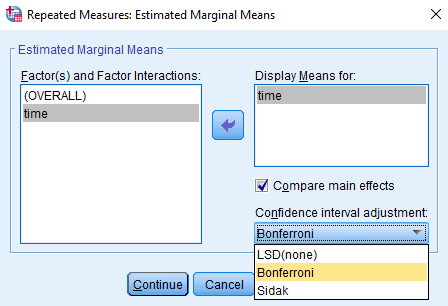Steps 10, 11 and 12 in running a one-way repeated measures ANOVA

14. Now click the ‘Options’ button on the right hand side.

15. Tick ‘Descriptive statistics’ and ‘Estimates of effect size’.

16. Click ‘Continue’.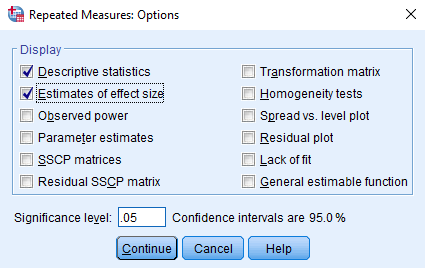Steps 14 and 15 in running a one-way repeated measures ANOVA

17. Then choose the ‘Plots’ button from the right.

18. Highlight your independent variable (time) and click the top blue button to move it into the ‘Horizontal Axis’ box.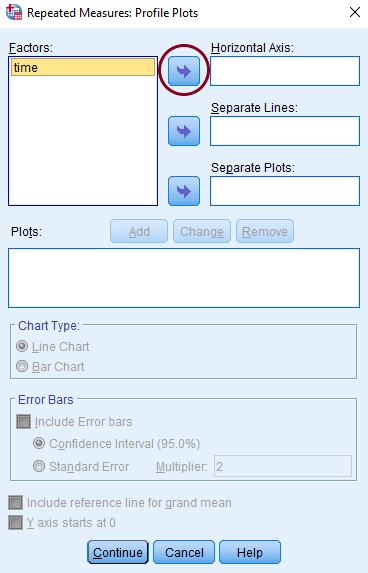Steps 17 and 18 in running a one-way repeated measures ANOVA

19. Click on ‘Add’ and then click ‘Continue’.

20. Then click ‘OK’.

This will open up the output window with your results.

## Interpreting the output and reporting in APA style:

The first thing to look at with this output is the ‘Mauchly’s Test of Sphericity’ table. This tests for the assumption of sphericity. For the purposes of this test we just want to look at the p-value in the ‘Sig’ column. If this is less than 0.05 then our data has violated the assumption of sphericity and we will use the Greenhouse-Geisser correction when we report our results. If it is greater than 0.05 then we can assume sphericity.

We could report this as follows:

Mauchly’s test of sphericity indicated that the assumption of sphericity had not been violated, χ²(2) = 0.497, p = 0.780.

Now we can look at our ‘Test of Within-Subjects Effects’ table and we can read from the ‘Sphericity Assumed’ row as explained above. We can see from this table that school year does have a significant effect on the students’ test scores because our p-value, 0.000, is less than 0.05. When our p-value is 0.000 we report it as p < 0.001 .

We could report our results as follows:

A one-way ANOVA with repeated measures showed that the school year of a student had a statistically significantly affects their test results, F(2) = 10.469, p < 0.001, η²ₚ = 0.428.

Because we found significance in our ANOVA we now want to look at some post hoc tests to find out where the differences lie. We look at the ‘Pairwise Comparisons’ table for the results of our Bonferroni-adjusted pairwise comparisons. We can see from this table that the students’ results were significantly higher in their second and third years compared to their first year however their results weren’t significantly different in their third year from their second year.

We could report our results as follows:

Bonferroni-adjusted pairwise comparisons showed that students’ test results in their second year (M = 57.38, SD = 5.579) and third year (M = 56.73, SD = 8.231) were higher than their results in their first year (M = 47.88, SD = 5.835). These improvements were statistically significant (p = 0.003 for both). However their third year scores were not significantly different from their second year scores (p = 1.000).

^back to contents

# 8. Running a Friedman test

A Friedman test is a non-parametric test that is used when we have a non-normal or ordinal dependent variable with two or more repeated measurements for our subjects. This is very similar to the one-way repeated measures ANOVA however our dependent variable doesn’t need to be normally distributed or continuous.

We will use the same example of comparing test scores taken over multiple years as we did for the one-way repeated measures ANOVA however this time we won’t assume that the scores are normally distributed.

Here’s how to run it in SPSS:

1. Select ‘Analyze’ from the top menu.
2. Choose ‘Nonparametric Tests’, ‘Legacy Dialogs’ and then ‘K Related Samples’.

3. Highlight all of your variables (in this example the three sets of test scores) and click on the blue button to move them into ‘Test Variables’.

4. Click on the ‘Statistics’ button and tick ‘Quartiles’.

5. Click ‘Continue’.

6. Make sure ‘Friedman’ is ticked in the ‘Test Type’ box.

7. Then click ‘OK’.

This will open up the output window with your results.

## Interpreting the output and reporting in APA style:

Looking at our ‘Test Statistics’ table we can see that the year of study had a significant effect on the students’ test scores because our p-value, 0.001, is less than 0.05.

We could report our results as follows:

A Friedman’s test showed that there was a significant difference between students’ test scores in their first year, second year and third year, χ²(2) = 14.533, p = 0.001 .

If the Friedman test is significant then we can run Wilcoxon signed-rank tests with Bonferroni adjustments to find out which of the groups are significantly different.

^back to contents

# 9. Running a Pearson’s correlation

A Pearson’s correlation is used to examine the relationship between two normally distributed, continuous variables. The Pearson’s correlation coefficient is a measure of the strength and direction of this relationship.

For example, we might want to see if there is a relationship between students’ test scores in English and Maths.

Here’s how to run it in SPSS:

1. Select ‘Analyze’ from the top menu.
2. Choose ‘Correlate’ and then ‘Bivariate’.

3. Select the variables you want to test (English scores and Maths scores) and click the button to move them into the ‘Variables’ box.

4. Click on the ‘Options’ button on the right hand side.

5. This is where you can ask SPSS to calculate some descriptive statistics if you haven’t already.

6. Click ‘Continue

7. Make sure ‘Pearson’ is ticked in the ‘Test of Significance’ box.

8. Then click ‘OK

This will open up the output window with your results.

## Interpreting the output and reporting in APA style:

Looking at our ‘Correlations’ table we can see that there is a significant relationship between the students’ English and Maths scores because our p-value, 0.000, is less than 0.05. When our p-value is 0.000 we report it as p<0.001.

We could report our results as follows:

We examined the relationship between students’ English and Maths scores. A Pearson correlation test showed that the two scores were significantly related, r = 0.659, p < 0.001, N = 51.

^back to contents

# 10. Running a Spearman’s rank test

A Spearman’s rank correlation is a non-parametric test used to examine the relationship between two ordinal variables. The Spearman’s correlation coefficient is a measure of the strength and direction of this relationship. This is similar to the Pearson’s correlation but our variables don’t need to be normally distributed or continuous.

We will use the same example of examining the relationship between test scores in English and Maths as we did for the Pearson’s correlation however this time we won’t assume that the scores are normally distributed.

Here’s how to run it in SPSS:

1. Select ‘Analyze’ from the top menu.
2. Choose ‘Correlate’ and then ‘Bivariate’.

3. Select the variables you want to test (English scores and Maths scores) and click the button to move them into the ‘Variables’ box.

4. Click on the ‘Options’ button on the right hand side.

5. This is where you can ask SPSS to calculate some descriptive statistics if you haven’t already.

6. Click ‘Continue

7. Make sure ‘Spearman’ is ticked in the ‘Test of Significance’ box.

8. Then click ‘OK

This will open up the output window with your results.

## Interpreting the output and reporting in APA style:

Looking at our ‘Correlations’ table we can see that there is a significant relationship between the students’ English and Maths scores because our p-value, 0.000, is less than 0.05. When our p-value is 0.000 we report it as p<0.001.

We could report our results as follows:

We examined the relationship between students’ English and Maths scores. A Spearman’s rho correlation test showed that the two scores were significantly related, rₛ = 0.651, p < 0.001, N = 51.

^back to contents

# 11. Running a simple linear regression

A simple linear regression is used to examine the linear relationship between one normally distributed, continuous predictor variable and one normally distributed, continuous outcome variable. For example, we might want to test whether a student’s English score predicts their Maths score.

Here’s how to run it in SPSS:

1. Select ‘Analyze’ from the top menu.
2. Choose ‘Regression’ and then ‘Linear’.

3. Highlight your dependent variable (Maths score) and click on the top blue arrow to move it to the ‘Dependent’ box.

4. Then highlight your independent variable (English score) and click on the second blue arrow to move it to the ‘Independent(s)’ box.

5. Click on the ‘Statistics’ button on the right hand side.

6. Tick ‘Collinearity diagnostics’ and ‘Durbin-Watson’.

7. Then click ‘Continue’.

8. Next click on ‘Plots’ on the right.

9. Highlight ‘*ZRESID’ and click on the top blue button to move it to ‘Y’.

10. Highlight ‘*ZPRED’ and click on the second blue button to move it to ‘X’.

11. Tick ‘Histogram’, ‘Normal probability plot’ and ‘Produce all partial plots’.

12. Click ‘Continue’ and then ‘OK’.

This will open up the output window with your results.

## Interpreting the output and reporting in APA style:

The first thing we want to do is check our assumptions. We should have already checked that the relationship between our variables is linear and that there are no significant outliers. We can next look at the Durbin-Watson statistic in our ‘Model Summary’ table. If this value is between 1.5 and 2.5 then we can say that our observations are independent and we have met the assumption.

We can then look at the scatterplot of the residuals. This should not have an obvious pattern and the points should be fairly evenly distributed over both the X and Y axis, much like the one above. If our plot looks something like this then we can say that our data is homoscedastic and we have met the assumption.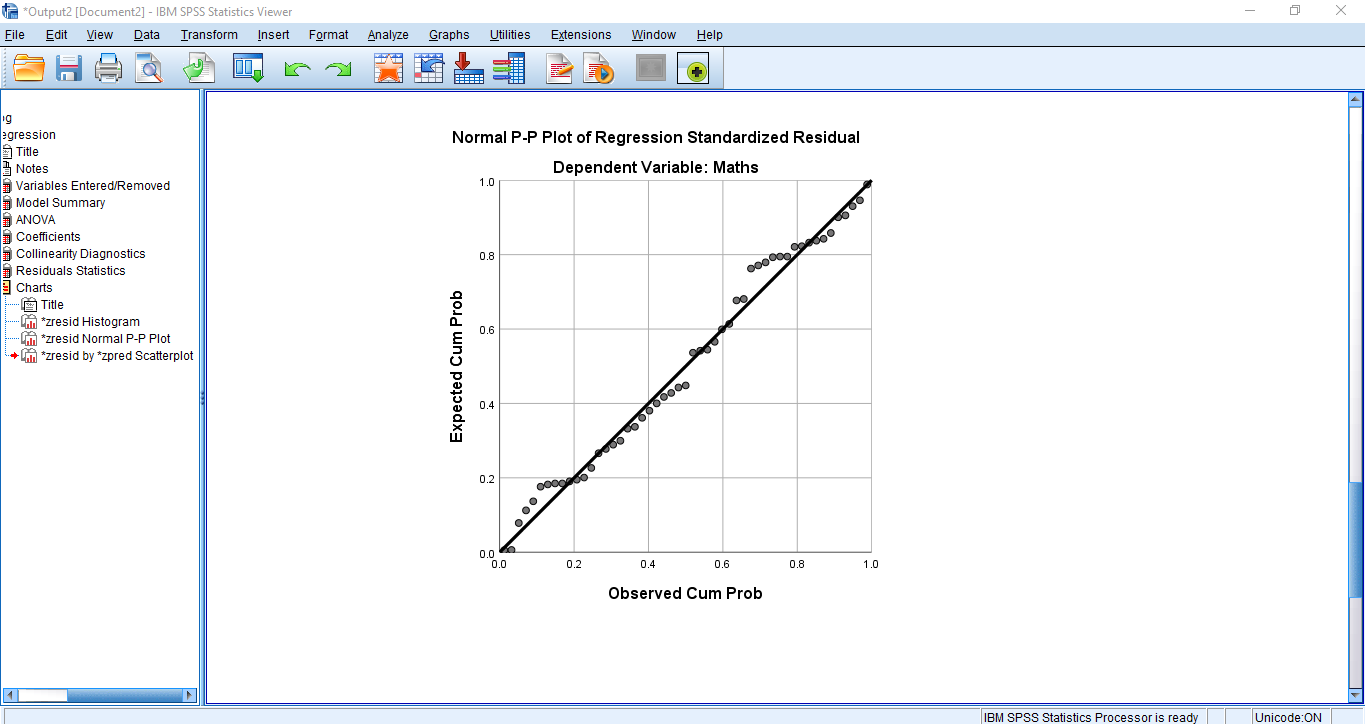Left: Histogram of the residuals with a normal curve overlaid; Right: A P-P plot of the residuals

Finally we need to check that the residuals are normally distributed. Either of the two plots above can be used for this. The histogram (on the left) should roughly match the normal curve that is overlaid in black and the points on the p-p plot (on the right) should be roughly along the straight line. In this example they are close enough so we can say that our residuals are normally distributed and our data meets this assumption.

Once we have checked that our data meets all the assumptions (and included that in the results section if needed) we can move on to looking at the results of our regression.

Looking at our ‘ANOVA’ table we can see that the students’ English score is a significant predictor of their Maths score in this model as our p-value, 0.000, is less than 0.05. When our p-value is 0.000 we report it as p<0.001.

We could report our results as follows:

A simple regression was used to predict a student’s Maths score from their score in an English test. Their English score explained a significant amount of the variance in their Maths score, F(1, 49) = 37.595, p < 0.001, R² = 0.434, R²adjusted = 0.423. The regression coefficient (B = 0.651) indicated that an increase of one point in a student’s English test corresponded, on average, to an increase of 0.651 points on their Maths test.

^back to contents

# 12. Running a multiple linear regression

A multiple linear regression is used to examine the linear relationship between multiple normally distributed, continuous predictor variables and one normally distributed, continuous outcome variable. For example, we might want to test whether a student’s English and Science scores predict their Maths score.

Here’s how to run it in SPSS:

1. Select ‘Analyze’ from the top menu.
2. Choose ‘Regression’ and then ‘Linear’.

3. Highlight your dependent variable (Maths score) and click on the top blue arrow to move it to the ‘Dependent’ box.

4. Then highlight your independent variables (English score and Science score) and click on the second blue arrow to move them to the ‘Independent(s)’ box.

5. Click on the ‘Statistics’ button on the right hand side.

6. Tick ‘Collinearity diagnostics’ and ‘Durbin-Watson’.

7. Then click ‘Continue’.

8. Next click on ‘Plots’ on the right.

9. Highlight ‘*ZRESID’ and click on the top blue button to move it to ‘Y’.

10. Highlight ‘*ZPRED’ and click on the second blue button to move it to ‘X’.

11. Tick ‘Histogram’, ‘Normal probability plot’ and ‘Produce all partial plots’.

12. Click ‘Continue’ and then ‘OK’.

This will open up the output window with your results.

## Interpreting the output and reporting in APA style:

The first thing we want to do is check our assumptions. We should have already checked that the relationship between our variables is linear and that there are no significant outliers. We can next look at the Durbin-Watson statistic in our ‘Model Summary’ table. If this value is between 1.5 and 2.5 then we can say that our observations are independent and we have met the assumption.

We can then look at the scatterplot of the residuals. This should not have an obvious pattern and the points should be fairly evenly distributed over both the X and Y axis, much like the one above. If our plot looks something like this then we can say that our data is homoscedastic and we have met the assumption.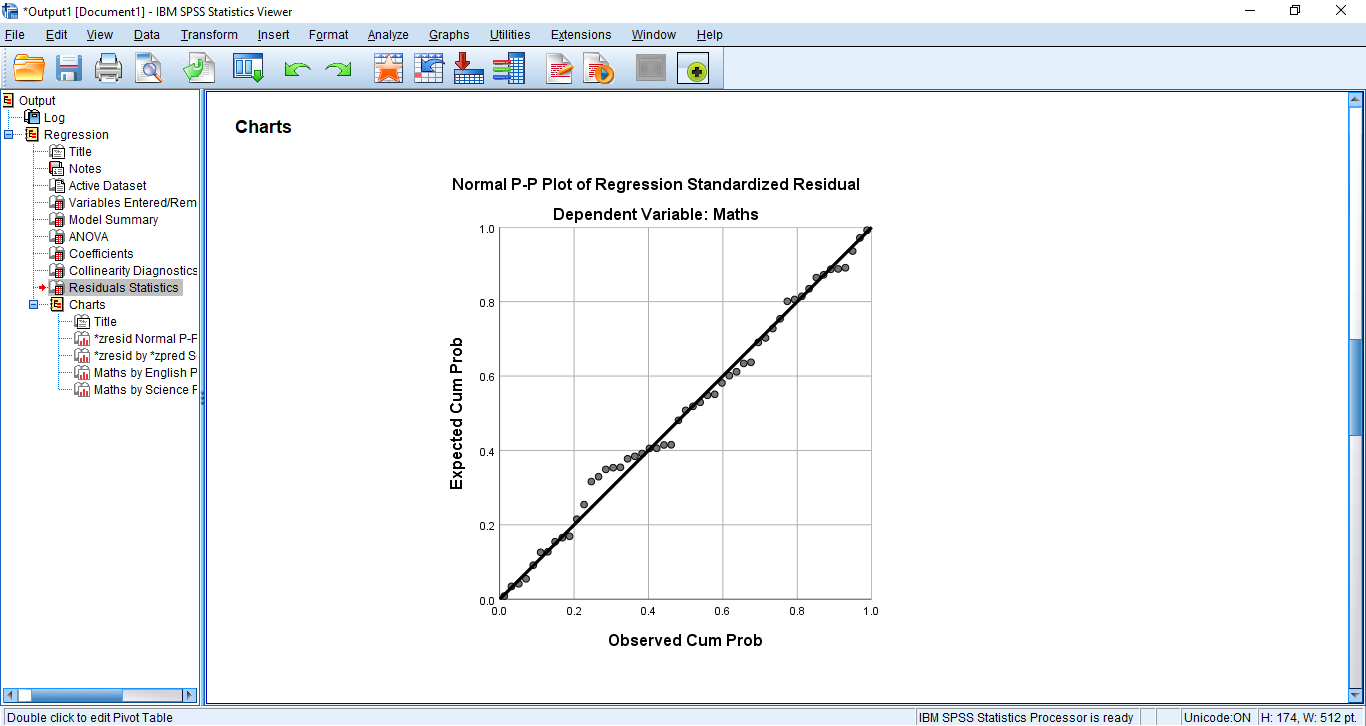Left: Histogram of the residuals with a normal curve overlaid; Right: A P-P plot of the residuals

Next we need to check that the residuals are normally distributed. Either of the two plots above can be used for this. The histogram (on the left) should roughly match the normal curve that is overlaid in black and the points on the p-p plot (on the right) should be roughly along the straight line. In this example they are close enough so we can say that our residuals are normally distributed and our data meets this assumption.

Finally we need to check multicollinearity. We can do this with the VIF values in our ‘Coefficients’ table. If these values are less than 10 then we have met this assumption.

Once we have checked that our data meets all the assumptions (and included that in the results section if needed) we can move on to looking at the results of our regression.

Looking at our ‘ANOVA’ table we can see that our overall regression model is a significant predictor of the students’ Maths scores in this model as our p-value, 0.000, is less than 0.05. When our p-value is 0.000 we report it as p<0.001. From looking at the ‘Coefficients’ table we can see that a student’s Science score is significant but their English score isn’t.

We could report our results as follows:

A multiple regression with enter method was used to predict a student’s Maths score from their score in an English test and their score in a Science test. The model explained a significant amount of the variance in their Maths score, F(2, 48) = 116.897, p < 0.001, R² = 0.830, R²adjusted = 0.823. Science score was a significant predictor of Maths score, β = 0.985, t(48) = 10.557, p < 0.001. An increase of one point in a student’s Science test corresponded, on average, to an increase of 0.959 points on their Maths test, B = 0.959. English score was not a significant predictor of Maths score, β = -0.99, t(48) = -1.063, p =0.293.

^back to contents

# 13. Running a chi-squared test

A chi-squared test is used to examine the relationship between two categorical variables. For example, testing to see if there is a relationship between gender and the mode of transport people use to get to work.

Here’s how to run it in SPSS:

1. Select ‘Analyze’ from the top menu.
2. Choose ‘Descriptive Statistics’ and then ‘Crosstabs’.

3. Highlight one of your variables (gender) and click on the top blue arrow to move it to the ‘Row(s)’ box.

4. Highlight your other variable (transport) and click on the second blue arrow to move it to the ‘Column(s)’ box.

5. Tick the ‘Display clustered bar charts’ option.

6. Select ‘Statistics’ on the right hand side.

7. Tick ‘Chi-square’ and ‘Phi and Cramer’s V’.

8. Then click ‘Continue’.

9. Select ‘Cells’ on the right.

10. Make sure ‘Observed’ is ticked in the ‘Counts’ section.

11. Tick ‘Row’, ‘Column’ and ‘Total’ in the ‘Percentages’ section.

12. Click ‘Continue’ and then ‘OK’.

This will open up the output window with your results.

## Interpreting the output and reporting in APA style:

The table and clustered bar chart above show us a nice visualisation of our data. They allow us to see the proportions of males and females that take each mode of transport.

Looking at our ‘Chi-Square Tests’ table we can see that there wasn’t a significant association between gender and mode of transport because our p-value, 0.235, is greater than 0.05.

We could report our results as follows:

A chi square test found that there was not a statistically significant association between gender and mode of transport, χ²(2) = 2.900, p = 0.235.

^back to contents

# Further Support

^back to contents

--

--